1800-1023-196

+91-120-4616500

# Rules for the determination of Oxidation number of an Atom

## Rules for the Determination of Oxidation Number of an Atom

The following rules are followed in ascertaining the oxidation number of an atom,

(i) If there is a covalent bond between two same atoms then oxidation numbers of these two atoms will be zero. Bonded electrons are symmetrically distributed between two atoms. Bonded atoms do not acquire any charge. So oxidation numbers of these two atoms are zero.

A: A or A – A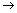A* + A*

For example, Oxidation number of Cl in Cl2, O in O2 and N in N2 is zero.

(ii) If covalent bond is between two different atoms then electrons are counted towards more electronegative atom. Thus oxidation number of more electronegative atom is negative and oxidation number of less electronegative atom is positive. Total number of charges on any element depends on number of bonds.

A – B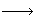A+ + B:

A – BA+2 +: B–2:

The oxidation number of less electronegative element (A) is + 1 and + 2 respectively.

(iii) If there is a coordinate bond between two atoms then oxidation number of donor atom will be + 2 and of acceptor atom will be – 2.

ABA2+ +:B-2 :

(iv) The oxidation number of all the atoms of different elements in their respective elementary states is taken to be zero. For example, in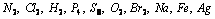etc. the oxidation number of each atom is zero.

(v) The oxidation number of a monoatomic ion is the same as the charge on it. For example, oxidation numbers of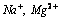and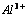ions are + 1, + 2 and + 3 respectively while those of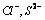and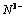ions are –1, –2 and –3 respectively.

(vi) The oxidation number of hydrogen is + 1 when combined with non-metals and is –1 when combined with active metals called metal hydrides such as LiH, KH, MgH2, CaH2 etc.

(vii) The oxidation number of oxygen is – 2 in most of its compounds, except in peroxides like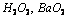etc. where it is –1. Another interesting exception is found in the compound OF2 (oxygen difluoride) where the oxidation number of oxygen is + 2. This is due to the fact that fluorine being the most electronegative element known has always an oxidation number of –1.

(viii) In compounds formed by union of metals with non-metals, the metal atoms will have positive oxidation numbers and the non-metals will have negative oxidation numbers.

For example,

(a) The oxidation number of alkali metals (Li, Na, K etc.) is always +1 and those of alkaline earth metals (Be, Mg, Ca etc) is + 2.

(b) The oxidation number of halogens (F, Cl, Br, I) is always –1 in metal halides such as KF, AlCl3, MgBr2, CdI2. etc.

(ix) In compounds formed by the union of different elements, the more electronegative atom will have negative oxidation number whereas the less electronegative atom will have positive oxidation number.

For example,

(a) N is given an oxidation number of –3 when it is bonded to less electronegative atom as in NH3 and NI3, but is given an oxidation number of + 3 when it is bonded to more electronegative atoms as in NCl3.

(b) Since fluorine is the most electronegative element known so its oxidation number is always –1 in its compounds i.e. oxides, interhalogen compounds etc.

(c) In interhalogen compounds of Cl, Br, and I; the more electronegative of the two halogens gets the oxidation number of –1. For example, in BrCl3, the oxidation number of Cl is –1 while that of Br is +3.

(x) For neutral molecule, the sum of the oxidation numbers of all the atoms is equal to zero. For example, in NH3 the sum of the oxidation numbers of nitrogen atom and 3 hydrogen atoms is equal to zero. For a complex ion, the sum of the oxidation numbers of all the atoms is equal to charge on the ion. For example, in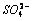ion, the sum of the oxidation numbers of sulphur atom and 4 oxygen atoms must be equal to –2.

(xi) It may be noted that oxidation number is also frequently called as oxidation state. For example, in H2O, the oxidation state of hydrogen is +1 and the oxidation state of oxygen is – 2. This means that oxidation number gives the oxidation state of an element in a compound.

(xii) In the case of representative elements, the highest oxidation number of an element is the same as its group number while highest negative oxidation number is equal to (8 – Group number) with negative sign with a few exceptions. The most common oxidation states of the representative elements are shown in the following table,

 Group Outer shell configuration Common oxidation numbers (states)except zero in free state I A ns1 +1 II A ns2 +2 III A ns2np1 +3, +1 IV A ns2np2 +4,+3,+2,+1, –1, –2, –3, –4 V A ns2np3 +5,+3,+1, –1, –3 VI A ns2np4 +6,+4,+2,–2 VII A ns2np5 +7,+5,+3, +1, –1

(xiii) Transition metals exhibit a large number of oxidation states due to involvement of (n –1) d electron besides ns electron.

(xiv) Oxidation number of a metal in carbonyl complex is always zero.

Example: Ni has zero oxidation state in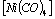.

(xv) Those compounds which have only C, H and O the oxidation number of carbon can be calculated by following formula,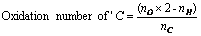Where,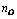is the number of oxygen atom,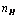is the number of hydrogen atom,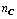is the number of carbon atom.

For example, (a)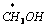;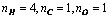Oxidation number of ‘C’ =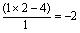(b)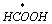;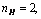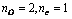Oxidation number of carbon =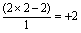## NEET & AIIMS Exam Sample Papers

 AIIMS SAMPLE PAPERS View More NEET SAMPLE PAPERS View More